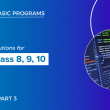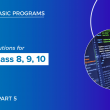# Qbasic programs solutions(part 4) for class 8, class 9 and class 10

Here, I have listed some of the qbasic programs. We also have mobile app where you can view all the qbasic programs. For better experience I highy recommend you to use our mobile app.

61  Write a program in qbasic to ask number and count total number of even digits

``````    CLS
INPUT "ENTER ANY NUMBER"; N
C = 0
WHILE N < > 0
R = N MOD 10
IF R MOD 2 = 0 THEN C = C + 1
N = N \ 10
WEND
PRINT "TOTAL NUMBER OF EVEN DIGITS"; C
END``````

62  Write a program in qbasic to generate the following series 5   55   555   5555   55555

``````CLS
A = 5
FOR I = 1 TO 5
PRINT A,
A = A * 10 + 5
NEXT I
END ``````

63  Write a program in qbasic to find the sum of all odd numbers from 1 to 100

``````CLS
FOR I = 1 TO 100 STEP 2
S = S + I
NEXT I
PRINT “SUM OF ALL ODD NUMBERS FROM 1 TO 100=”; S
END  ``````

64  Write a program in qbasic to generate the series 2   22   222………13th term

``````CLS
A# = 2
FOR I = 1 TO 13
PRINT A#;
A# = A# * 10 + 2
NEXT I
END  ``````

65  Write a program in qbasic to print all natural numbers from 1 to 100 in descending order

``````CLS
FOR I = 100 TO 1 STEP - 1
PRINT I,
NEXT I
END ``````

66  Write a program in qbasic to enter any ten strings and sort in ascending order

``````CLS
DIM N(10) AS STRING
FOR I = 1 TO 10
INPUT "ENTER THE STRINGS"; N(I)
NEXT I
FOR I = 1 TO 10
FOR J = 1 TO 10 - I
IF N(J) > N(J + 1) THEN SWAP N(J), N(J + 1)
NEXT J
NEXT I
PRINT "STRINGS ARRANGED IN ASCENDING ORDER"
FOR I = 1 TO 10
PRINT N(I)
NEXT I
END ``````

67  Write a program in qbasic to print sum of square of any two ask numbers

``````CLS
INPUT “ENTER FIRST NUMBER”; A
INPUT “ENTER SECOND NUMBER”; B
S = A ^ 2 + B ^ 2
PRINT “SUM OF SQUARE OF TWO NUMBERS ”; S
END
﻿``````

68  Write a program in qbasic to print all even numbers from 2 to 100 also print its sum

``````REM PROGRAM TO DISPLAY print all even number from 2 to 100

CLS
FOR I = 2 TO 100 STEP 2
PRINT I,
S = S + I
NEXT I
PRINT “SUM OF ALL EVEN NUMBERS FROM 1 TO 100=”; S
END  ``````

69  Write a program in qbasic to ask number and find sum of cube of digits

``````    CLS
INPUT "ENTER ANY NUMBER"; N
S = 0
WHILE N <> 0
R = N MOD 10
S = S + R ^ 3
N = N \ 10
WEND
PRINT "SUM OF CUBE OF DIGITS"; S
END ``````

70   Write a program in qbasic to ask number and find sum of odd digits

``````    CLS
INPUT "ENTER ANY NUMBER"; N
S = 0
WHILE N <> 0
R = N MOD 10
IF R MOD 2 = 1 THEN S = S + R
N = N \ 10
WEND
PRINT "SUM OF ODD DIGITS"; S
END ``````

71  Write a program in qbasic to enter any 10 numbers and find the sum of numbers

``````    CLS
FOR I = 1 TO 10
INPUT "ENTER THE NUMBERS"; N(I)
S = S + N(I)
NEXT I
PRINT "SUM OF 10 NUMBERS"; S
END ``````

72  Write a program in qbasic TO ENTER ANY DIGIT AND PRINT CUBE OF ODD DIGITS

``````CLS
INPUT "ENTER ANY NUMBER"; N
S = 0
WHILE N <> 0
R = N MOD 10
IF R MOD 2 = 1 THEN S = S + R ^ 3
N = N \ 10
WEND
PRINT "SUM OF CUBE OF ODD DIGITS"; S
END ``````

73  Write a program in qbasic to enter any ten strings and print the longest strings

``````    CLS
INPUT "ENTER FIRST STRING"; A\$
FOR I = 2 TO 10
INPUT "ENTER NEXT STRING"; B\$
IF LEN(B\$) > LEN(A\$) THEN A\$ = B\$
NEXT I
PRINT "LONGEST STRING="; A\$
END
﻿``````

74  Write a program in qbasic to generate the series 0.00003, 0.0003, 0.003, 0.03, 0.3

``````    CLS
A = .00003
FOR I = 1 TO 5
PRINT A
A = A * 10
NEXT I
END
﻿``````

75  Write a program in qbasic to ask any number and print the prime factorial of a given number

``````
CLS
INPUT "ENTER ANY NUMBER"; N
F = 1
FOR I = 1 TO N
C = 0
FOR J = 1 TO I
IF I MOD J = 0 THEN C = C + 1
NEXT J
IF C = 2 THEN F = F * I
NEXT I
PRINT "PRIME FACTORIAL ="; F
END
﻿``````

76  Write a program to enter any 10 numbers and display the smallest one.

``````    CLS
INPUT "ENTER FIRST NUMBER"; N
FOR I = 2 TO 10
INPUT "ENTER NEXT NUMBER"; S
IF S <  N THEN N = S
NEXT I
PRINT “THE SMALLEST NUMBER IS “; N
END
﻿``````

77  Write a program in qbasic to ask any number and check whether the given number is palindrome or not

``````    CLS
INPUT "ENTER ANY NUMBER"; N
A = N
S = 0
WHILE N < > 0
R = N MOD 10
S = S * 10 + R
N = N \ 10
WEND
IF A = S THEN
PRINT A; "IS PALINDROME"
ELSE
PRINT A; "IS NOT PALINDROME"
END IF
END ``````

78  Write a program in qbasic to ask three sides of a triangle and determine whether a triangle can be formed or not

``````<!-- wp:code {"canvasClassName":"cnvs-block-core-code-1606732332679"} -->
<pre class="wp-block-code"><code class="">    CLS
INPUT “ENTER THREE SIDES OF A TRIANGLE”; A,B,C
IF (A + B) > C AND (B + C) > A AND (A + C) > B THEN
PRINT “THE TRIANGLE CAN BE FORMED”
ELSE
PRINT “THE TRIANGLE CANNOT  BE FORMED”
END IF
END
</code></pre>
<!-- /wp:code -->``````

79  Write a program in qbasic to ask three sides of a triangle and determine whether a triangle is equilateral isosceles or scalene triangle or not

``````    CLS
INPUT “ENTER THREE SIDES OF A TRIANGLE”; A,B,C
IF A = B AND B = C THEN
PRINT “IT IS A EQUILATERAL TRIANGLE”
ELSEIF A = B OR B = C OR C = A THEN
PRINT “IT IS ISOSCELES TRIANGLE”
ELSEIF A < > B AND B < > C THEN
PRINT “ IT IS A SCALENE TRIANGLE”
END IF
END ``````

80  Write a program in qbasic to generate the series 10   20   30………100

``````    CLS
A = 1
FOR I = 10 TO 100 STEP 10
PRINT I,
NEXT I
END  ``````

##### Previous Post## Qbasic programs solutions(part 3) for class 8, class 9 and class 10

##### Next Post## Qbasic programs solutions(part 5) for class 8, class 9 and class 10

##### Related Posts## Graphics Programming in QBASIC

Getting Started with QBASIC Graphics QBASIC’s graphics commands were straightforward yet powerful, allowing users to create impressive visuals.…## Basic Commands in QBASIC

Welcome to the world of QBASIC programming! If you’re new to coding or just starting out, QBASIC is…## QBASIC for Educational Purposes

Introduction In today’s fast-paced digital world, programming has become an essential skill for both students and professionals. However,…